Next: Problem Up: Local Star Formation Process Previous: Protostellar Evolution of Supercritical   Contents

# Accretion Rate

Using equation (2.26), the necessary time for a mass-shell at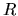to reach the center (free-fall time) is expressed as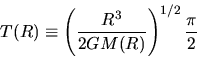(4.79)

(for detail of this section see Ogino, Tomisaka, & Nakamura 1999).

Consider two shells whose initial radii areand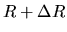. The time difference for these two shells to reach the center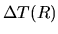can be written down using equation (4.79) as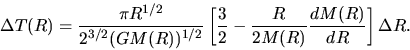(4.80)

Mass in the shell betweenand,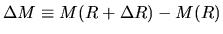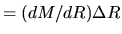, accretes on the central object in. Thus, mass accretion rate for a pressure-free cloud is expressed as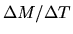. This leads to the expression as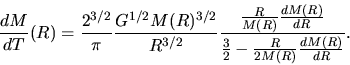(4.81)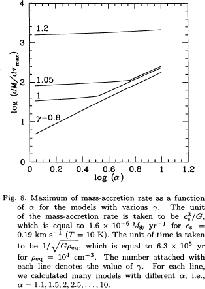This gives time variation of the accretion rate. Consider two clouds with the same density distribution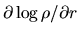but different absolute value. Since these two clouds have the same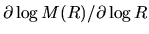, the mass accretion rate depends only on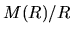, and is expressed as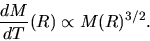(4.82)

This indicates that the accretion rate is proportional to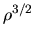, while the time scale is to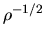. This is confirmed by hydrodynamical simulations of spherical symmetric isothermal clouds (Ogino et al.1999). When the initial density distribution is the SIS as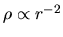, the mass included insideis proportional to radius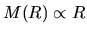. In this case, equation (4.81) gives a constant accretion rate in time. In Figure 4.8 we plot the mass accretion rate against the cloud density.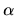represents the cloud density relative to that of a hydrostatic Bonnor-Ebert sphere. This shows clearly that the mass accretion rate is proportional to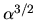for massive clouds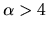. This is natural since the assumption of pressure-less is valid only for a massive cloud in which the gravity force is predominant against the pressure force.

Similar discussion has been done by Henriksen, André, & Bontemps (1997) to explain a decline in the accretion rate from Class 0 to Class I IR objects. They assumed initial density distribution of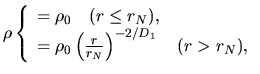(4.83)

as shown in Figure 4.9. Since the free-fall-time of the gas contained in the inner core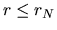is the same, such gas reaches the center once. It makes a very large accretion rate at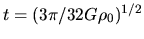as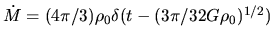. If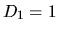,for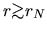. Since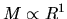and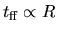, equation(4.81) predicts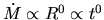. A constant accretion rate is expected for this power-law and the accretion rate is converged to a constant value after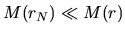. If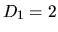,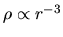for. Since for this power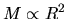and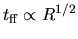, equation(4.81) predicts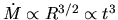. They gave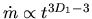for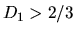.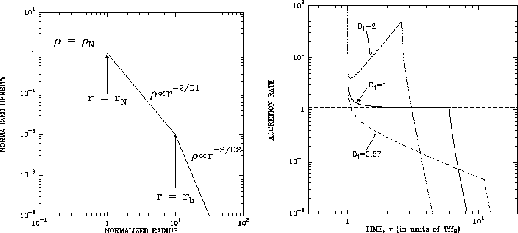SubsectionsNext: Problem Up: Local Star Formation Process Previous: Protostellar Evolution of Supercritical   Contents
Kohji Tomisaka 2007-07-08